# Assignments

In the assignments below exponential equations must be solved. It is allowed to use logarithms, see also Logarithmic functions and graphs, but we prefer to find an equation with two exponential functions with the same base and solve it. Sometimes this requires calculations with exponents, so it is important to master this topic, see also Rules for exponents.

1. Solve: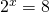Solution

2. Solve:Solution

3. Solve: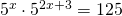Solution

4. Solve: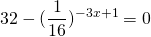Solution

5. Solve: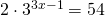Solution

6. Solve: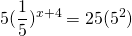Solution

7. Solve: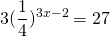Solution

8. Solve: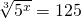Solution

9. Solve: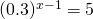Solution

10. Solve: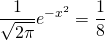Solution

0